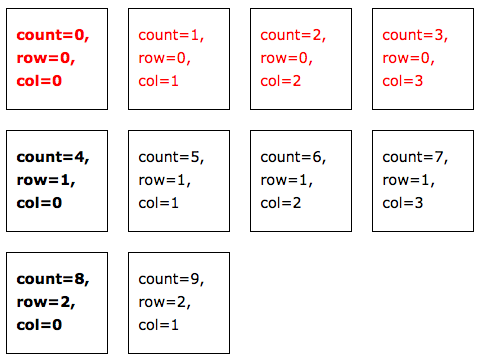# Create a grid layout using adi_calc

Here we want to produce a grid layout using the counting capabilities of adi_calc and with plenty of styling hooks. We’ll be using the `reset` and `reset_counter` attributes in version 1.1 of the plugin.

### The Tools

• Textpattern 4.0.7+### The Code

In the code below, we’re:

1. Explicitly initialising the counters & in true programming tradition everything starts at zero
2. Looping through a series of articles
3. For each article, create some markup & display the counter values
``````<!-- initialise variables -->
<txp:variable name="count" value="0" />
<txp:variable name="row" value="0" />
<txp:variable name="col" value="0" />
```
```<div class="adi_calc">
```
```	<!-- loop though a series of articles -->
<txp:article_custom section="articles">
```
```		<!-- create HTML id based on overall article count -->
<txp:variable name="id">article<txp:variable name="count" /></txp:variable>
```
```		<!-- create class based on row & column -->
<txp:variable name="class">row<txp:variable name="row" /> col<txp:variable name="col" /></txp:variable>
```
```		<!-- output article with computed id & class -->
<div id="<txp:variable name="id" />" class="<txp:variable name="class" />">
<p>count=<txp:variable name="count" />, row=<txp:variable name="row" />, col=<txp:variable name="col" /></p>
<txp:body />
</div>
```
```		<!-- increment article counter -->
```
```		<!-- increment column counter, resetting to zero when get to 4, count resets (i.e. rows) -->
```
```	</txp:article_custom>
```
```</div>
``````

Notes:

• the wrapping `<div>` is there to make life simple & obvious
• the counter values are displayed in the output for demonstration purposes only
• the example articles don’t actually have any bodies to output!
• the number of columns can be adjusted very easily, simply by changing the reset value in the last call of adi_calc
• use `<txp:if_last_article>` if you want to do something special with the last article (#9 in the example)

### The Styling

This will produce the example output.

``````.adi_calc {
overflow:hidden;
}
```
```.adi_calc div { /* style each article */
width:100px;
height:100px;
float:left;
margin:10px;
border:1px solid black
}
```
```.adi_calc div.row0 { /* style first row */
color:red;
}
```
```.adi_calc div.col0 { /* style first column */
clear:left;
font-weight:bold;
}
``````

The above example is simply a demonstration but the possibilities are endless. For example, in real life you might want to set the number of columns as a variable for use in the `reset` and also to identify the last column (i.e. when column count = number of columns – 1). Or you could even implement alternating effects (zebra stripes?) using smd_if with it’s `divisible` operator.